# Teaching Decision Support Systems using Spreadsheets

### Prof. Abdel-Aziz Mohamed

California State University-Northridge

## Introduction

The management science field has evolved for over 50 years and is now a mature field within the broad category of applied mathematics. Many of the management science algorithms are however very complex mathematically and cannot be understood by managerial users. However with the power of the Microsoft Excel macro language, Visual Basic for Applications (VBA), those algorithms can be made accessible to non-experts by providing them with simplified input screens and output reports. Users no longer need to understand all of the technical details behind the algorithms. If one can teach the power of spreadsheet modeling to management science students in quantitative analysis and decision support systems classes, students will view those algorithms as something useful.

This paper illustrates the effectiveness of using Excel spreadsheets with VBA to teach an undergraduate course in Decision Support Systems (DSS). Several examples are presented to show how one can make management science algorithms accessible to non-experts. The paper shows how to manipulate the Excel Solver with a VBA program to solve a specific business problem. Detailed explanations of how the VBA code is developed are also presented. The DSS course is offered to undergraduate students at California State University-Northridge. Most of the students who take the course major in either management science or management information systems. The students who take the course must take three prerequisite courses in database, quantitative analysis and operations management as well as pass the upper division writing proficiency exam. The course covers the components and the development methodologies for building decision support systems. Two group projects are required: 1) an analysis of a current DSS and 2) development of a spreadsheet-based DSS.

The aim of the first project is to familiarize students with some existing DSS. Each group is required to present and demonstrate a DSS in class. DSS News (Vol. 1, No. 10) presents several examples of DSS software that can be downloaded from the Internet. DSS News is a free Internet newsletter published by D. J. Power.

For the second project, each group selects an application area and goes through the process of developing a specific model-driven DSS. Students are asked to develop a user friendly decision support system that can be used by non-experts. The next four sections illustrate specific model-driven DSS that have been developed for car loan analysis, blending analysis, stock portfolio analysis and selection, and site location analysis problems.

## Car Loan Analysis Problem

This first example shows how to develop a simple spreadsheet-based DSS that can be used by people who have no prior experience with computers to determine the monthly payment for a car loan. The code is designed to ask the user to input the loan size, the number of years and the annual interest rate. Then the code calls the Excel PMT function to calculate the monthly payment and it displays the result. The only thing needed from the user is to click on the button given on the spreadsheet to start the VBA code and respond to the series of questions. The users will neither see the code nor the way the code calculates the monthly payment. Figure 1 shows the Excel worksheet and the dialogue box for the car loan application. The following VBA code is used to generate all of the dialogue boxes and call the Excel PMT function to calculate the monthly payment:

Sub carloan()
Dim loanamount As Currency, rate As Double, numberyears As Double,
Dim numbermonths As Double, Monthlypayment As Currency
MsgBox ("CAR LOAN APPLICATION")
loanamount = InputBox("What is the loan amount?")
rate = InputBox("What is the interest rate (%)?")
rate = rate / 12
rate = rate / 100
numberyears = InputBox("How many years?")
numbermonths = numberyears * 12
Monthlypayment = Pmt(rate, numbermonths, - loanamount, 0)
MsgBox "The monthly payment is " & Format(Monthlypayment, "\$0,000") & "per month"
End Sub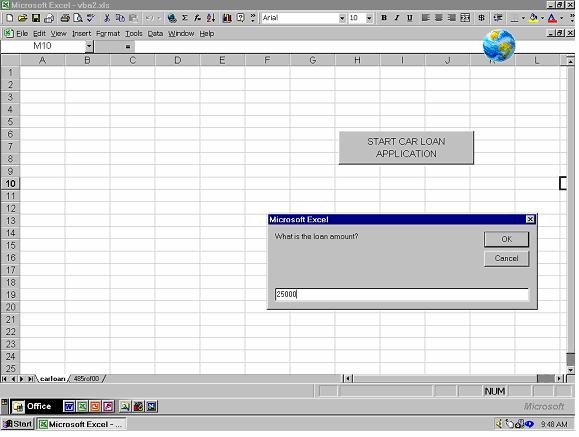Figure 1: DSS for the Car Loan Problem

## A Blending Problem

This model-driven DSS is developed for a company that provides customers with custom feed mixing. The DSS determines the amount of feed of each type required to fill an order with minimum cost and satisfy the ingredient requirments. Data about the order size as well as ingredients must be provided. The original problem is found in Ragsdale (2001, Page 80). Table 1 gives the composition of each feed type and its cost per pound.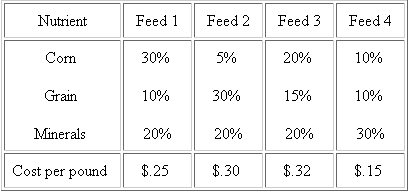The decision variables, Xi, were defined to be the amount of feed type i, i = 1, 2, 3, 4. Assume that the order size is 8000 pounds of feed and the percentages of corn, grain and minerals needed are 20%, 15% and 15%, respectively. The objective is to fill the customer order at the lowest possible cost. The objective function and the model are expressed as:

Minimize .25X1 + .3X2 + .32X3 + .15X4

Subject to:

X1 + X2 + X3 + X4 = 8000

(.3X1 + .05X2 + .2X3 + .1X4)/( X1 + X2 + X3 + X4 ) ³ .20

(.1X1 + .3X2 + .15X3 + .1X4)/( X1 + X2 + X3 + X4 ) ³ .15

(.2X1 + .2X2 + .2X3 + .3X4)/( X1 + X2 + X3 + X4 ) ³ .15

A decision support system was developed to solve problems of this type. The user needs to input the amount of feed ordered as well as the percentage of corn, grain, and minerals that need to be included in the feed. Then, the user clicks on the button labeled "START BLENDING APPLICATION" and Excel provides the user with the amounts of feed type 1, 2, 3 and 4 that should be mixed together to fill the order at the lowest possible cost. Figure 2 shows the input screen and the output reports for this application.

The VBA code calls the Excel Solver function to solve the LP model developed for this problem. The Excel Solver is an add-in that is developed by Frontline Systems, Inc., not by Microsoft. For help about the Solver add-in, visit the Frontline Systems� web site at www.frontsys.com/mlvbaref.htm. The subroutine blending is the only VBA code written for this problem. The functions most often used to manipulate the Excel Solver add-in are SolverReset, SolverOk, SolverAdd, SolverOptions, and SolverSolve. The SolverReset function resets all buttons to clear all previous settings. The SolverOk identifies the target cell; whether the problem is minimization or maximization, and the cells that will contain the output values. The SolverAdd function is used to add one constraint to the model. Therefore, the SolverAdd function is used as many times as the number of constraints is in the problem. The SolverOptions identifies the options in the Excel Solver such as, assume linear, assume nonneg, etc. The SolverSolve function provides the solution to the problem. After solving the problem, if the variable result in the code has been assigned a value of 5, the problem will not have a feasible solution. Figure 2 shows the input screen and output report designed for this problem.

Sub blending()
solverReset
solverOk Setcell:=Range("b23"), maxminval:=2, Bychange:= range("b19:b22")
Solveroptions assumeLinear:=True, AssumeNonneg:=True
result = SolverSolve(Userfinish:=True)
If result = 5 MsgBox "There exist no feasible solutions."
End Sub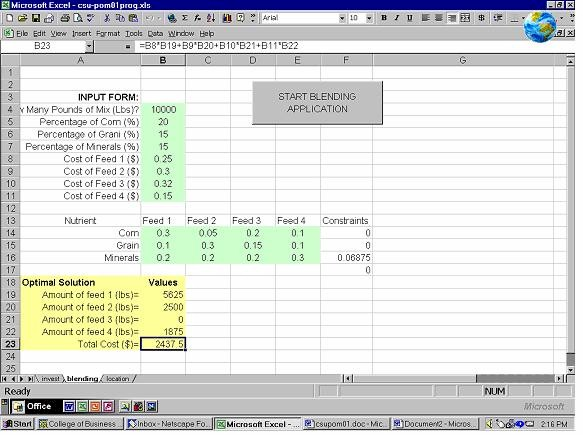Figure 2: Model-driven DSS for a Blending Problem

## Portfolio Selection Problem

This DSS involves determining the optimal proportions and amounts of funds to be invested in the stocks A, B, and C that minimize the risk and meet a prespecified annual rate of return. This problem is also found in Ragsdale (2001, pages 368-369). The following are the annual returns for the past 12 years for stocks A, B and C:
 Year Stock A Stock B Stock C 1 2 3 4 5 6 7 8 9 10 11 12 11.2% 10.8% 11.6% -1.6% -4.1% 8.6% 6.8% 11.9% 12% 8.3% 6% 10.2% 8% 9.2% 6.6% 18.5% 7.4% 13% 22% 14% 20.5% 14% 19% 9% 10.9% 22% 37.9% -11.8% 12.9% -7.5% 9.3% 48.7% -1.9% 19.1% -3.4% 43% Averages 7.64% 13.43% 14.93%

Excel is used to determine the average annual returns and to generate the following covariance matrix.
 A B C A .000258 -.00025 .00440 B -.00025 .00257 -.00542 C .00440 -.00542 .03877

The decision variables, pi, are defined as the proportion of the fund invested in stock i, for i = 1, 2 and 3. The objective function that will be minimized is the portfolio variance. One constraint is used to restrict the annual return of the investment to at least 12%. The mathematical model is: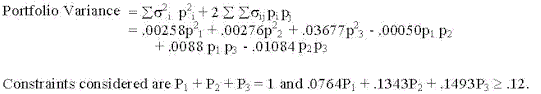Excel solver add-in was manipulated through the VBA code, Sub Portfolio, to determine the optimal solution to the previous Nonlinear Programming (NLP) problem. In Subroutine SolverOptions, assumelinear was set to "False" since the model used is nonlinear.

Sub Portfolio()
solverReset
solverOk Setcell:=Range("g13"), maxminval:=2, _
Bychange:=Union(Range("b23"), Range("b24"), Range("b25"))
Solveroptions assumeLinear:=False, AssumeNonneg:=True
SolverSolve Userfinish:=True
End Sub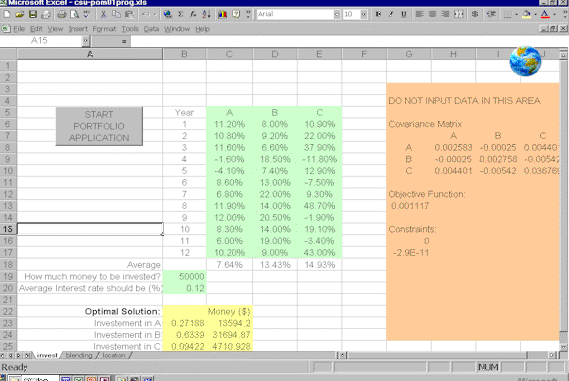Figure 3: DSS for the Portfolio Selection Problem

## Location Problem

The following DSS was developed to determine the optimal facility location that minimizes the Eucliden distance. The following single facility location problem is found in Fitzsimmons and Fitzsimmons (1998, page 170). The problem is modeled as a non-constraint NLP problem. The distances between customer locations and x and y coordinates as well as the weights assigned to customer locations are:

 Customer Location x-coordinate y-coordinate #orders 1 2 3 4 1 2 3 4 2 3 5 1 7 1 3 5

The following is the NLP formulation:

Minimize (7). sqrt{(1-x1)^2 +(2-y1)^2} + sqrt{(2-x1)^2 +(3-y1)^2} + (3) sqrt{(3-x1)^2 +(5-y1)^2} + (5)sqrt{(4-x1)^2 +(1-y1)^2} Figure 4 presents a model-driven DSS developed to solve this problem. The distances between four customer locations and the x and y coordinates are supplied by the users. Subroutine location is the VBA code. The only thing the code does is call the Solver add-in. There is no need to use the SolverAdd function this time since we have no constraints. The user has to input the distances between each customer and the x and y coordinates and Excel will give the optimal location of the facility.

Sub location()
solverReset
solverOk Setcell:=Range("a24"), maxminval:=2, _
Bychange:=Union(Range("b17"), Range("c17"))
Solveroptions assumeLinear:=False, AssumeNonneg:=True
SolverSolve Userfinish:=True
End Sub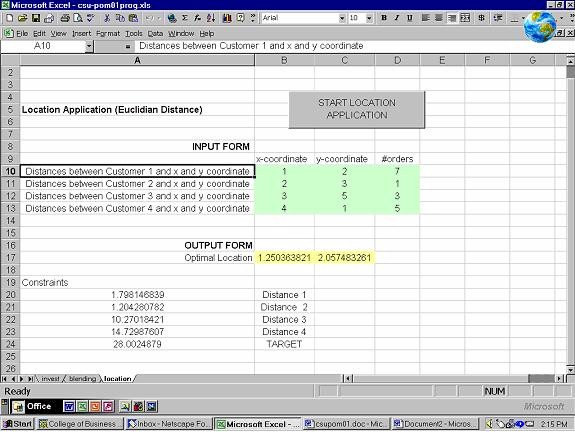Figure 4: Model-Driven DSS for the Location Problem

## Conclusions and Recommendation

My experience leads me to highly recommended using spreadsheets in management science and DSS courses. In general, students react with great enthusiasm to each new spreadsheet feature they learn. Students should learn how to convert management science algorithms into practical applications that help them in their own field of study. Student interest in learning about spreadsheet-based DSS results not just from the excitement of the new techniques but also from the many opportunities they have to perform analyses that they are convinced are much like what they will face after graduation. When teaching DSS, professors should consider meeting in computer labs and consider giving regular spreadsheet class assignments.

## References

Albright, S. Christian (2001), VBA Modelers Developing Decision Support Systems with Microsoft Excel, Duxbury.

Fitzsimmons, J. and Fitzsimmons, M. (1988), Service Management: Operations, Strategy, and Information Technology, Second Edition, McGraw Hill.

Ragsdale, C.(2001), Spreadsheet Modeling and Decision Analysis, Southwestern Thomson Learning.

Turban, E. and Aronson, J (2001), Decision Support Systems and Intelligent Systems, Prentice Hall.

Winston, W. and Albright, S.C. (2001), Practical Management Science, Second Edition, Duxbury.

Prof. Abdel-Aziz Mohamed, Department of Systems and Operations Management, California State University-Northridge, 18111 Nordhoff Street, Northridge, CA 91330-8378, (818) 677-4644, E-mail: amohamed@csun.edu. A version of this paper originally appeared in the Proceedings of the 13th Annual CSU-POM Conference, California State University, San Bernardino, February 23-24, 2001.

### Citation

Mohamed, Abdel-Aziz. "Teaching Decision Support Systems using Spreadsheets," DSSResources.COM, 03/14/2003.

Abdel-Aziz Mohamed provided permission to publish this article and feature it at DSSResources.COM on Saturday, November 16, 2002. This article was posted at DSSResources.COM on March 14, 2003.